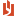## Practice problems and final exam problems

 Author Message NEASSupreme BeingGroup: Administrators Posts: 4.2K, Visits: 1.2K Practice problems and final exam problems Cryer and Chan present the theory of ARIMA modeling and derive the relevant equations. They explain how to model actual time series, and they provide illustrations. End of chapter exercises give numerical examples of the algebraic equations, but solutions are not in the textbook.  Candidates need worked exercises to understand the material. The discussion forum has practice problems showing how the theory is applied. The final exam problems are like the practice problems, though they are multiple choice questions. If you can solve the practice problems on the discussion forum, you can answer the final exam questions.     AttachmentsPractice problems and final exam problems.pdf (2.5K views, 15.00 KB) cjh411Forum NewbieGroup: Awaiting Activation Posts: 2, Visits: 15 I just bought the Kindle version of the book, and the practice problems don't appear to line up with what is in the book. Are the problems from an old edition, or are they supposed to be different?[NEAS: The practice problems are geared to multiple choice exams; the textbook problems are more theoretical. For the exams, focus on the practice problems; the textbook explains the concepts needed to solve them.] Edited 6 Years Ago by NEAS
##### Merge Selected
Merge into selected topic...

Merge into merge target...

Merge into a specific topic ID...